• 2：pandas.DataFrame.fillna¶ 利用各行/各列的均值去填充空值   以列为例，简单来说，填充时指定各列填充的值为各列的均值就好了。对行的操作，要注意操作时参数要改成行。 values = dict([(col_name, col_mean) ...
利用各行/各列的均值去填充该行/列的空值
参考： 1：python—创建字典的方式 2：pandas.DataFrame.fillna¶
利用各行/各列的均值去填充空值
以列为例，简单来说，填充时指定各列填充的值为各列的均值就好了。对行的操作，要注意操作时参数要改成行。
values = dict([(col_name, col_mean) for col_name, col_mean in zip(group.columns.tolist(), group.mean().tolist())]) # 参看1，生成字典，key为列名，value为列对应的均值
group.fillna(value=values, inplace=True) # 参看2，填充空值，value这里选择为字典形式，字典的key指明列，字典的value指明填充该列所用的值


一个例子
现在有如下含有空值的数据：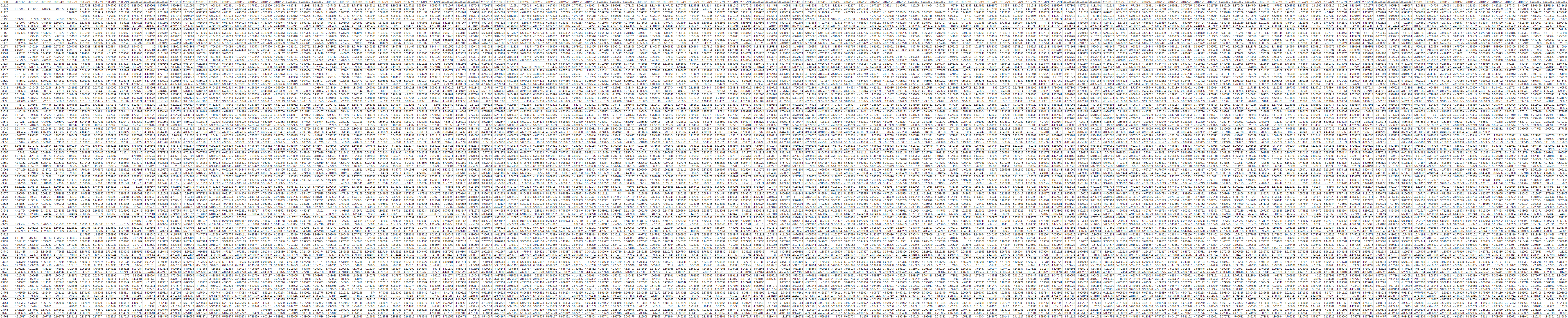数据下载： 链接：https://pan.baidu.com/s/1FiRFHhSsuspIdBIIUEXJ2w 提取码：0a4b

该数据中，有92个站点(行)，各站点有120个月(列)，存在若干站点在某些月的数据有缺失——在数据上表现为空值。现在我们希望填充空值，对于单个站点用相同月份的均值去填充该月份。比如：G1120的2009年1月缺失，填充时应该用G1120在2010 -2018历年1月的均值填充它。   解决思路：   1、将原数据wind_df按照月份分组，得到12个月组；   2、对每个月组，利用列均值去填充空值；   3、将填充后的月组合并成新的Dataframe：filled_df；   4、对filled_df按照时间顺序进行排列，得到和元数据相同的格式；   以下是代码：
def fill_NAN():
filePath = r'G:\Shenzhen-wind\code for paper\EOF\wind_df.csv'
wind_df = pd.read_csv(filePath, engine='python', index_col=, encoding='utf-8-sig')

# 这几行非核心代码，可删去不要，放在这里可以显示哪些列有缺失，
a = wind_df.isnull().any()
print('---')
print(a[a==True]) # 打印有缺失的列
print('---')

wind_df = wind_df.T # 先转置原始数据，方便操作
wind_df.index =pd.to_datetime(wind_df.index) # 将时间列（也是索引列）转换为时间格式
groups = wind_df.groupby(wind_df.index.month) # 按照时间列的月份对原数据分组
filled_df = pd.DataFrame() # 新的Dataframe：filled_df，用于合并所有已填充完毕的月组
for mon, group in groups: # 遍历月组
print(mon) # 月组名
# print(group.mean()) # 默认得到的是各列的均值
values = dict([(col_name, col_mean) for col_name, col_mean in zip(group.columns.tolist(), group.mean().tolist())]) # 参看1，生成字典，key为列名，value为列对应的均值
group.fillna(value=values, inplace=True) # 参看2，填充空值，value这里选择为字典形式，字典的key指明列，字典的value指明填充该列所用的值
# print(group)
filled_df = filled_df.append(group) # 将填充后的月组合并到filled_df中

filled_df.sort_index(inplace=True) # index是时间格式，这里sort_index方法根据时间先后对其排序
filled_df = filled_df.T # 转置回来，保证与原数据格式一致
filePath = r'G:\Shenzhen-wind\code for paper\EOF\filled_wind_df.csv'
filled_df.to_csv(filePath, encoding='utf-8-sig') # 存下该数据

if __name__ == '__main__':
fill_NAN()


展开全文• 参考：pandas找出重复行后取均值并合并 import pandas as pd import numpy as np import matplotlib as mpl %matplotlib inline from ggplot import * theme_bw() ggplot麻烦的很，内部用的是老pandas的东西，比如...
参考：pandas找出重复行后取均值并合并
import pandas as pd
import numpy as np
import matplotlib as mpl
%matplotlib inline

from ggplot import *
theme_bw()

ggplot麻烦的很，内部用的是老pandas的东西，比如sort之类的，还有一个date啥啥也有问题。如果要解决只能手动修改py文件。
import numpy as np

import pymongo,pandas as pd
from bson import ObjectId

import matplotlib as mb
import matplotlib.pyplot as plt
from matplotlib.backends.backend_pdf import PdfPages
import plotnine as p9

from textblob import TextBlob
from textblob.sentiments import NaiveBayesAnalyzer

from dateutil import parser

from ggplot import *
from pandas.plotting import register_matplotlib_converters
register_matplotlib_converters()

导入数据
pcfr = pd.read_excel('hair_dryer.xlsx')
df = pcfr
# '=='后面替换品牌名字即可
m = df[df['product_title']=='remington ac2015 t|studio salon collection pearl ceramic hair dryer, deep purple']
m = df

情感分析函数
中间 df[‘review_date’] = pd.to_datetime(df[‘review_date’])的时候会有玄学报错……整表和按品牌分类后的方法不一样……
def s_c_f(df):

# 去重
df.duplicated().value_counts()

# NaN remove
df['review_body'].str.split(expand = True)

# date format convert
# 切表用这个
# df['review_date'] = df.review_date.apply(lambda x:parser.parse(x))
# 整表用这个
df['review_date'] = pd.to_datetime(df['review_date'])

#将date设置为index
df = df.set_index('review_date')

## sentiment analysis
# func for polarity
def sentiment_calc(text):
try:
return TextBlob(text).sentiment.polarity
except:
return None

# func for subjectivity
def sentiment_calc_sub(text):
try:
return TextBlob(text).sentiment.subjectivity
except:
return None

df['polarity'] = df['review_body'].apply(sentiment_calc)
df['subjectivity'] = df['review_body'].apply(sentiment_calc_sub)

return df

画图函数
只画polarity
def drawfig_polarity(result):
# 在s_t_f中，通过set_index，把日期设为index,就可以用这种方式求年平均值
#m = result.groupby(result.index.year).mean()

'''reset_index by default does not modify the DataFrame;
it returns a new DataFrame with the reset index.
If you want to modify the original,
use the inplace argument: df.reset_index(drop=True, inplace=True).
Alternatively, assign the result of reset_index by doing df = df.reset_index(drop=True).'''
new = result.reset_index()

plot = ggplot(aes(x='review_date', y='polarity'), data=new) + \
geom_point() + \
geom_line(color = 'blue') + \
stat_smooth(span = 0.1)

return plot

三个都画上去
def drawfig_star(df):

# 在s_t_f中，通过set_index，把日期设为index,就可以用这种方式求年平均值
#d = result.groupby(result.index.year).mean()#求完其实自动删除了不是数值的列

'''reset_index by default does not modify the DataFrame;
it returns a new DataFrame with the reset index.
If you want to modify the original,
use the inplace argument: df.reset_index(drop=True, inplace=True).
Alternatively, assign the result of reset_index by doing df = df.reset_index(drop=True).'''

# 统一尺度
#df['subjectivity'] = df['subjectivity'].map(lambda x: x*45*0.76*0.8*0.7*0.4*1.08)
#df['polarity'] = df['polarity'].map(lambda x: x*45*0.76*0.8*0.7)

def normalize(data):
return (data - data.mean()) / data.std()

#     df['subjectivity'] = df['subjectivity'].map(lambda x: normalize(x))
df['subjectivity'] = normalize(df['subjectivity'])
#     df['polarity'] = df['polarity'].map(lambda x: normalize(x))
df['polarity'] = normalize(df['polarity'])
df['star_rating'] = normalize(df['star_rating'])

#ggplot
df['x'] = df.index
df = pd.melt(df, id_vars='x')

plot = ggplot(aes(x='x', y='value', color='variable'), df) + \
geom_point() + stat_smooth(span = 0.1) + geom_line()

plot.make()
#     plot.fig.set_size_inches(30, 5, forward=True)
#     plot.fig.set_dpi(100)
#     plot.fig

return plot

result = s_c_f(m)

把没必要的去掉。😂队友洗完的数据里有precentage,persentage,percentage，最开始报错搞好久。
# 在s_t_f中，通过set_index，把日期设为index,就可以用这种方式求年平均值
# d = result.groupby(result.index.day).mean()#求完其实自动删除了不是数值的列
#d

resultdf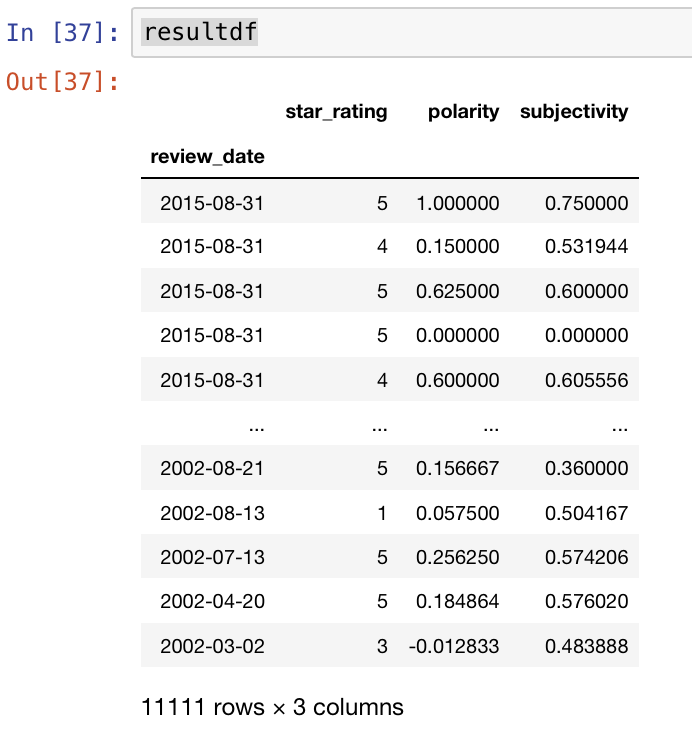重头戏：根据日取平均
我们有好多数据，每天的条数不一样，我想每天只有一条，怎么取平均去重再放回去我想了好久，其实方法好简单。
# raw_df=resultdf.groupby(resultdf.index.day).mean()
# raw_df = resultdf.groupby([resultdf.index.year,resultdf.index.month]).mean()
raw_df = resultdf.groupby([resultdf.index.year.rename('year'), resultdf.index.month.rename('month')]).mean()

这时候我们已经完成了重复日期取平均放回去的去重。但是怎么处理前面的year,month是另一个问题。现在长这个样子。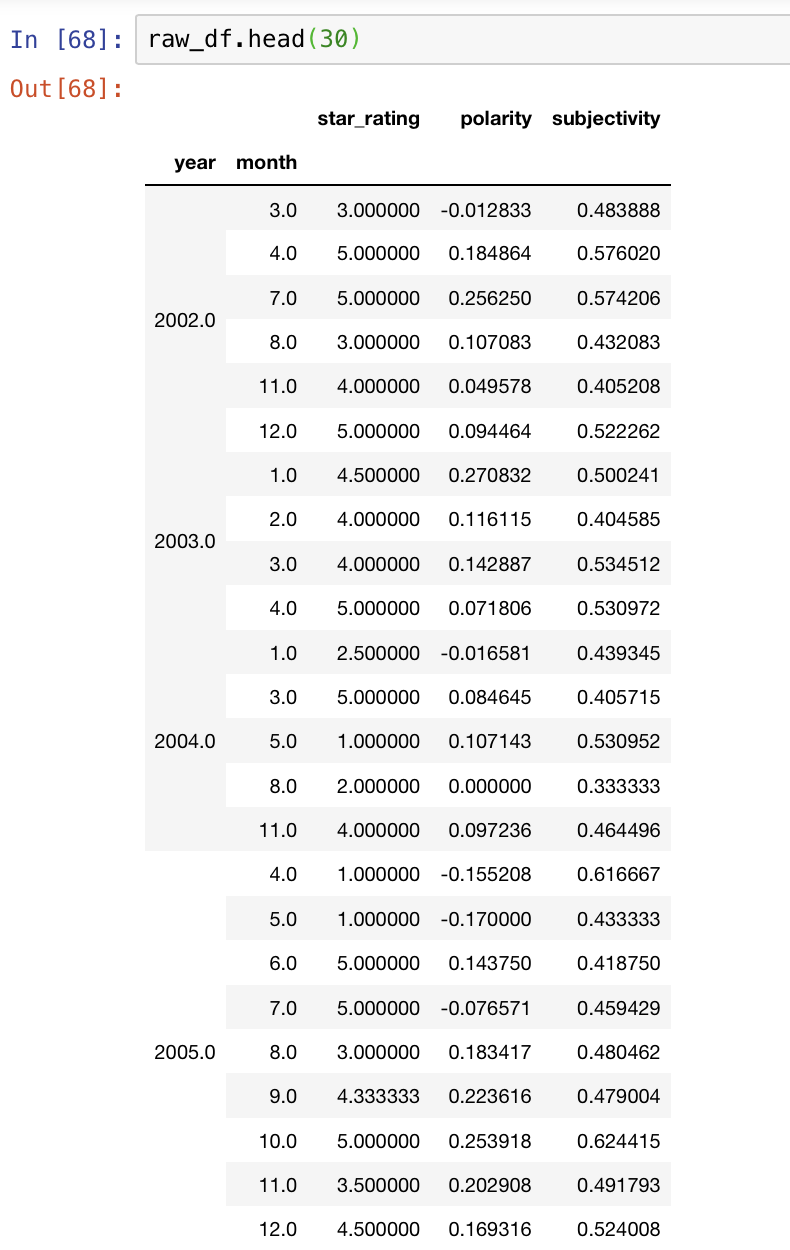先把他们放回去。注意不管是set_index（）还是reset_index()操作，本身不改变，要用另一个变量接住。 同时新建一列day。
raw_df = raw_df.reset_index()
raw_df['day']=1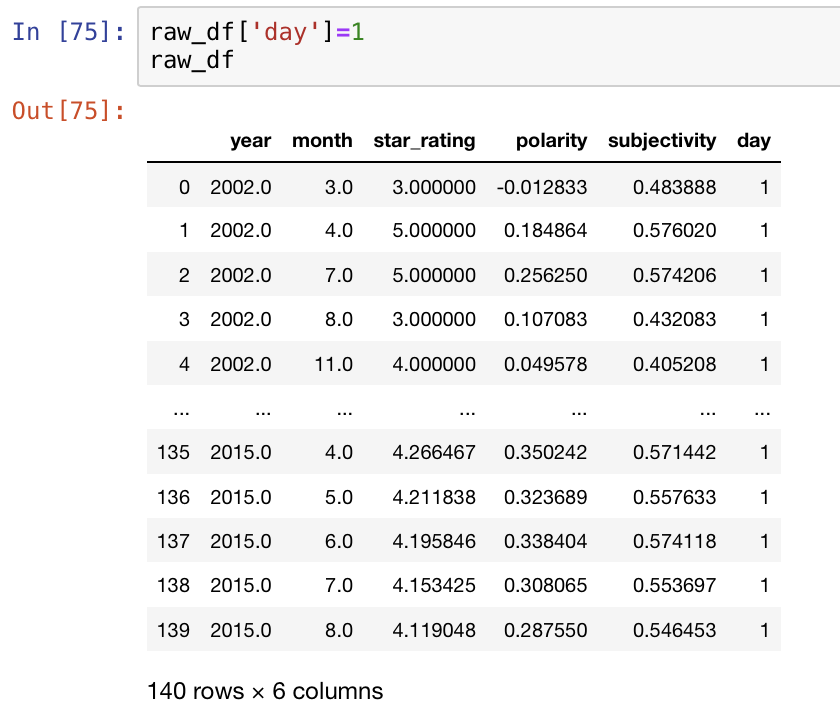一个非常美貌的函数。生成date_time格式的日期。但是必须得3列。所以我们前面才会新搞一个day出来。
from datetime import datetime
# df['review_date'] = df.apply(lambda row: datetime(row['year'], row['month']), axis=1)
raw_df['review_date'] = pd.to_datetime(raw_df[['year','month','day']])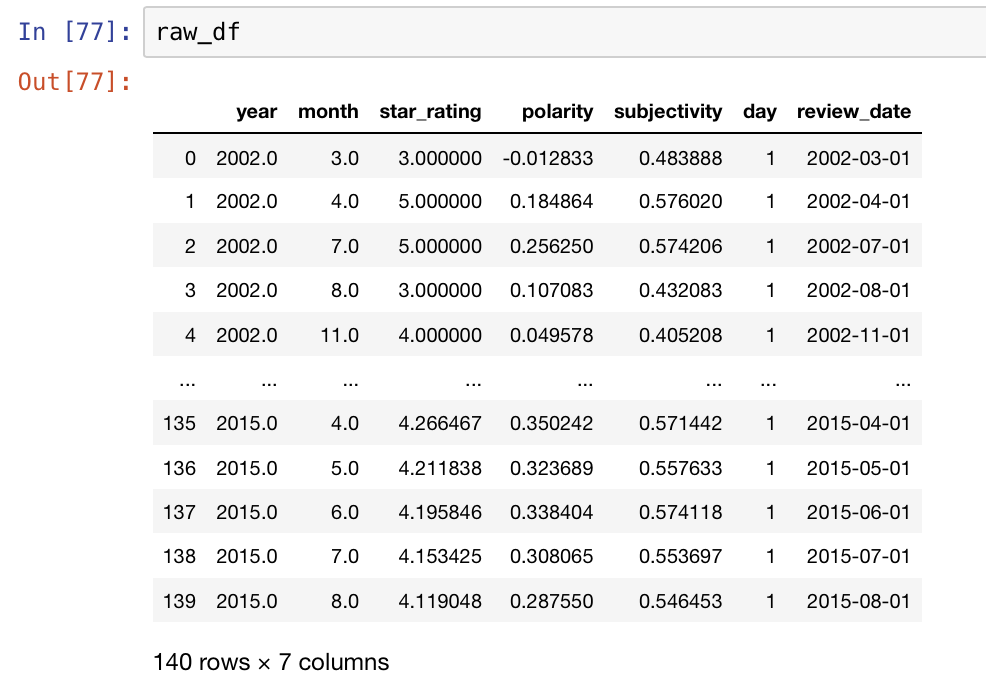可有可无的一步。
raw_df.sort_index(ascending=False,inplace=True)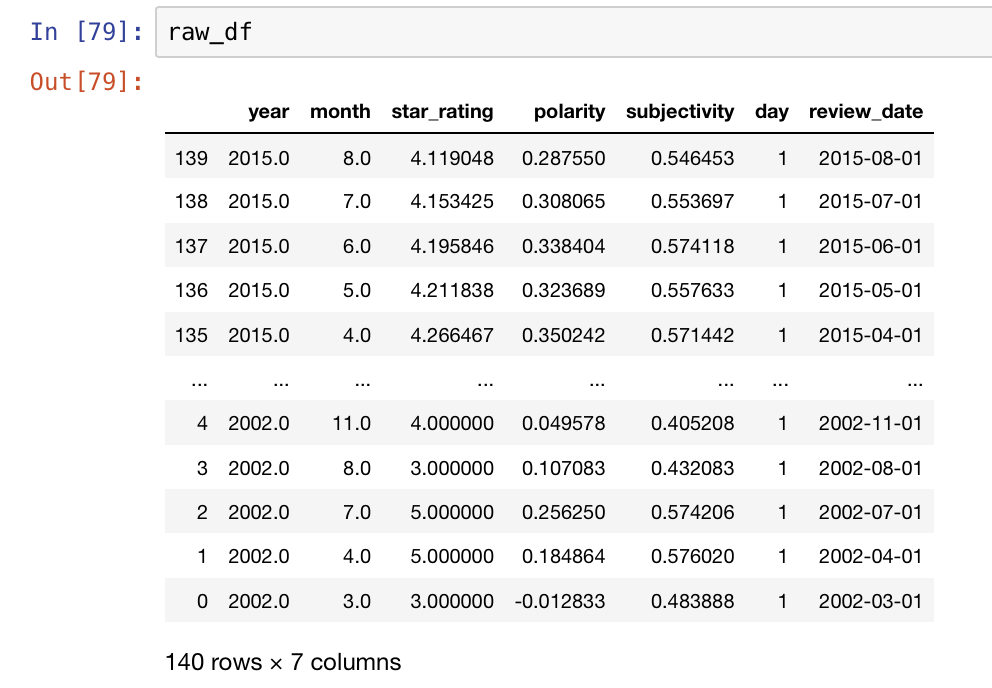把之前没用的扔掉。
raw_df = raw_df.drop(['year','month','day'], axis=1)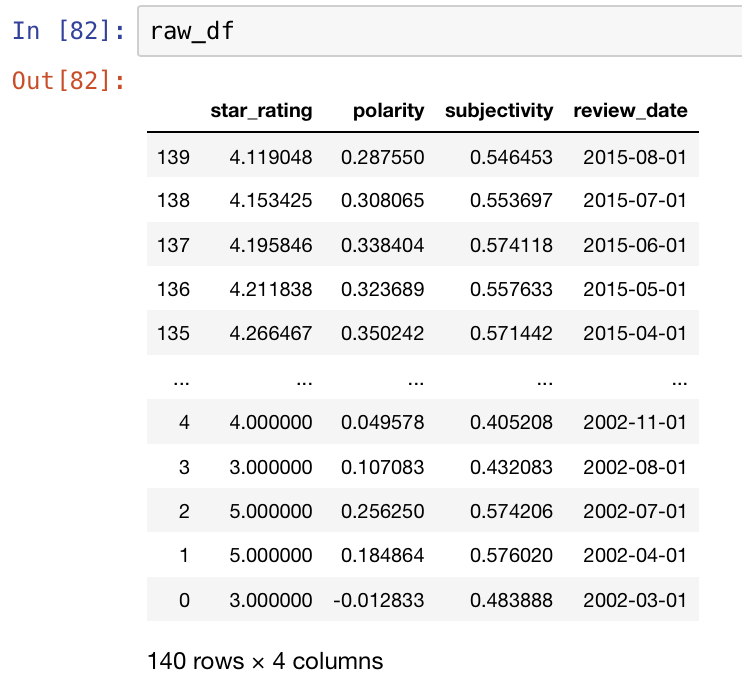raw_df = raw_df.set_index('review_date')
raw_df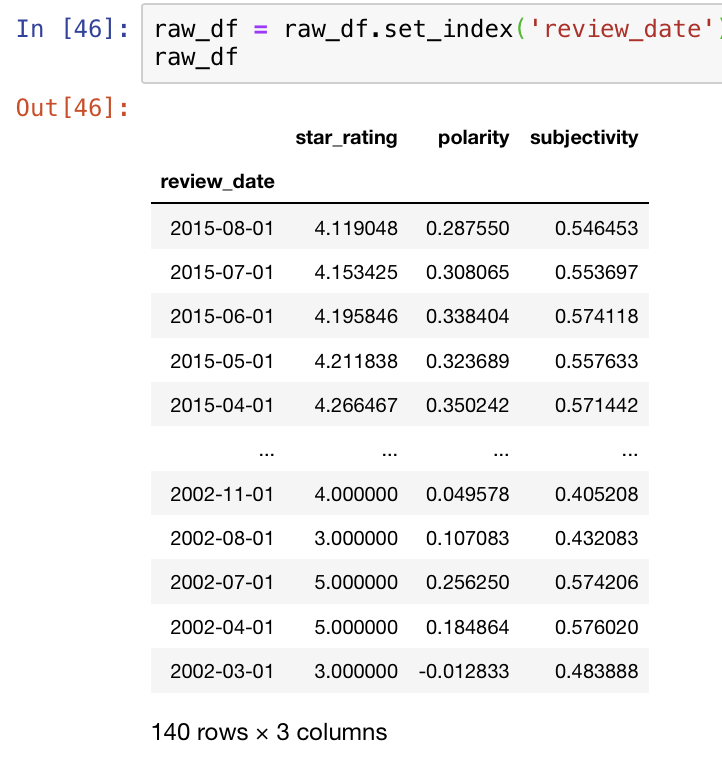我们的问题被完美解决～可以开始画图了
plot1 = drawfig_polarity(raw_df)
plot1.save(filename = 'new_plot')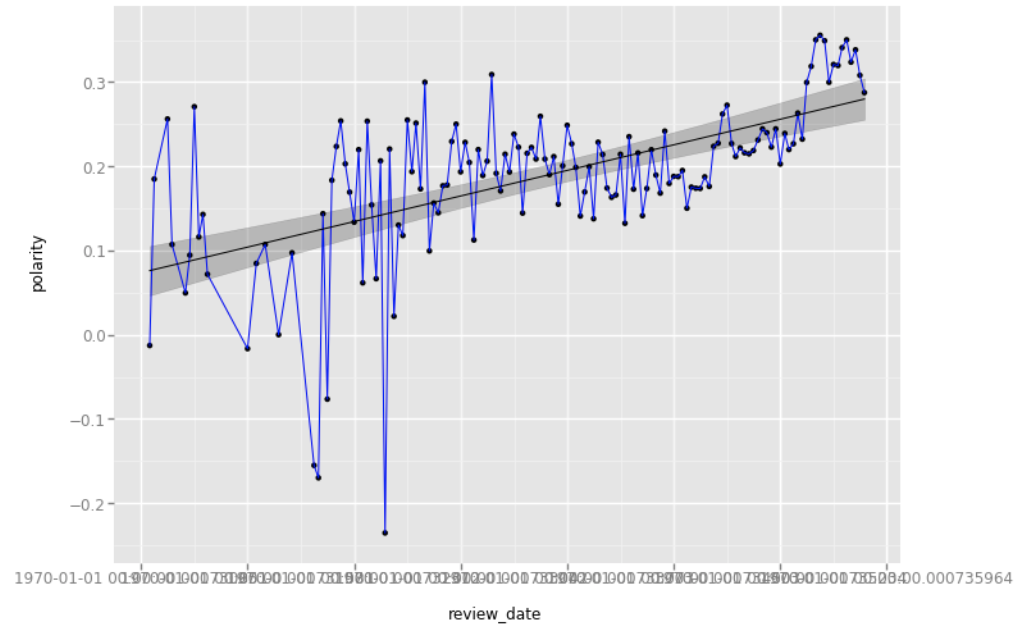#将date设置为index
plot2 = drawfig_star(raw_df)
#ggsave(filename="new_plot2.jpg", width=20, height=4, units='in', plot=plot2)
#plot2.save(filename='new_plot2')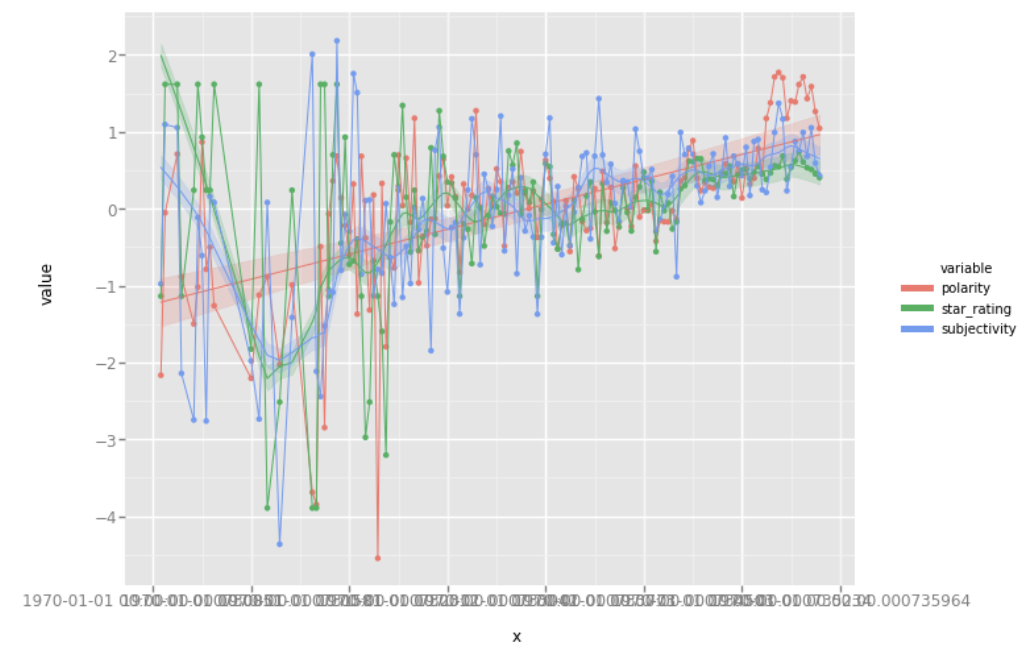展开全文python
•python 数据处理 特征工程
• ## Pandas

2019-10-02 12:39:46
Pandas 什么是Pandas pandas能干什么 怎么用pandas Series DataFrame 时间对象处理 数据分组和聚合 其他常用方法 1、什么是Pandas ​ 当大家谈论到数据分析时，提及最多的语言就是Python和SQL，而Python之所以...

Pandas
什么是Pandaspandas能干什么怎么用pandas
SeriesDataFrame时间对象处理数据分组和聚合其他常用方法
1、什么是Pandas
​ 当大家谈论到数据分析时，提及最多的语言就是Python和SQL，而Python之所以适合做数据分析，就是因为他有很多强大的第三方库来协助，pandas就是其中之一，它是基于Numpy构建的，正因pandas的出现，让Python语言也成为使用最广泛而且强大的数据分析环境之一。如果说没有pandas的出现，目前的金融数据分析领域还应该是R语言的天下。2、Pandas能干什么
Pandas的主要功能：
具备对应其功能的数据结构DataFrame，Series集成时间序列功能提供丰富的数学运算和操作灵活处理缺失数据.....
以上就是pandas能完成的一些基础操作，当然并不完全，下面就来看看pandas到底是怎么用的。3、怎么用Pandas
安装方法：

pip install pandas

引用方法：

import pandas as pd

3.1、Series
Series是一种类似于一维数组的对象，由一组数据和一组与之相关的数据标签(索引)组成。在数据分析的过程中非常常用。3.1.1、创建方法
第一种：
pd.Series([4,5,6,7,8])
执行结果：
0    4
1    5
2    6
3    7
4    8
dtype: int64
# 将数组索引以及数组的值打印出来，索引在左，值在右，由于没有为数据指定索引，于是会自动创建一个0到N-1（N为数据的长度）的整数型索引，取值的时候可以通过索引取值，跟之前学过的数组和列表一样
-----------------------------------------------
第二种：
pd.Series([4,5,6,7,8],index=['a','b','c','d','e'])
执行结果：
a    4
b    5
c    6
d    7
e    8
dtype: int64
# 自定义索引，index是一个索引列表，里面包含的是字符串，依然可以通过默认索引取值。
-----------------------------------------------
第三种：
pd.Series({"a":1,"b":2})
执行结果：
a    1
b    2
dtype: int64
# 指定索引
-----------------------------------------------
第四种：
pd.Series(0,index=['a','b','c'])
执行结果：
a    0
b    0
c    0
dtype: int64
# 创建一个值都是0的数组
-----------------------------------------------
​ 对于Series，其实我们可以认为它是一个长度固定且有序的字典，因为它的索引和数据是按位置进行匹配的，像我们会使用字典的上下文，就肯定也会使用Series3.1.2、缺失数据
dropna() # 过滤掉值为NaN的行fill() # 填充缺失数据isnull() # 返回布尔数组，缺失值对应为Truenotnull() # 返回布尔数组，缺失值对应为False
# 第一步，创建一个字典，通过Series方式创建一个Series对象

st = {"sean":18,"yang":19,"bella":20,"cloud":21}
obj = pd.Series(st)
obj
运行结果：
sean     18
yang     19
bella    20
cloud    21
dtype: int64
------------------------------------------
# 第二步
a = {'sean','yang','cloud','rocky'}  # 定义一个索引变量
------------------------------------------
#第三步
obj1 = pd.Series(st,index=a)
obj1  # 将第二步定义的a变量作为索引传入

# 运行结果：
rocky     NaN
cloud    21.0
sean     18.0
yang     19.0
dtype: float64
# 因为rocky没有出现在st的键中，所以返回的是缺失值
通过上面的代码演示，对于缺失值已经有了一个简单的了解，接下来就来看看如何判断缺失值
1、
obj1.isnull()  # 是缺失值返回Ture
运行结果：
rocky     True
cloud    False
sean     False
yang     False
dtype: bool

2、
obj1.notnull()  # 不是缺失值返回Ture
运行结果：
rocky    False
cloud     True
sean      True
yang      True
dtype: bool

3、过滤缺失值 # 布尔型索引
obj1[obj1.notnull()]
运行结果：
cloud    21.0
yang     19.0
sean     18.0
dtype: float643.1.3、Series特性
import numpy as np
import pandas as pd
从ndarray创建Series:Series(arr)
arr = np.arange(10)

sr = pd.Series(arr)  # ndarray创建Series
与标量（数字）进行运算：sr * 2
srx = sr * 2  # 与标量（数字）进行运算
两个Series运算
sr * srx  # 两个Series运算
布尔值过滤：sr[sr>0]
sr[sr>3]  # 布尔值过滤
统计函数：mean()、sum()、cumsum()
# 统计函数
sr.mean()
sr.sum()
sr.cumsum()3.1.4、支持字典的特性
从字典创建Series：Series(dic),
dic = {"A":1,"B":2,"C":3,"D":4,"E":5}

# 字典创建Series
dic_arr = pd.Series(dic)  
In运算：'a'in sr、for x in sr
"A" in dic_arr
------------------------------
for i in dic_arr:
print(i)
键索引：sr['a'],sr[['a','b','d']]
dic_arr[['A','B']]  # 键索引
键切片：sr['a':'c']
dic_arr['A':'C']  # 键切片
其他函数：get('a',default=0)等
dic_arr.get("A",default=0)3.1.5、整数索引
pandas当中的整数索引对象可能会让初次接触它的人很懵逼，接下来通过代码演示：
sr = pd.Series(np.arange(10))
sr1 = sr[3:].copy()
sr1
运行结果：
3    3
4    4
5    5
6    6
7    7
8    8
9    9
dtype: int32
# 到这里会发现很正常，一点问题都没有，可是当使用整数索引取值的时候就会出现问题了。因为在pandas当中使用整数索引取值是优先以标签解释的，而不是下标
sr1
解决方法：
loc属性 # 以标签解释iloc属性 # 以下标解释
sr1.iloc  # 以下标解释

sr1.loc  # 以标签解释3.1.6、Series数据对齐
pandas在运算时，会按索引进行对齐然后计算。如果存在不同的索引，则结果的索引是两个操作数索引的并集。
sr1 = pd.Series([12,23,34], index=['c','a','d'])
sr2 = pd.Series([11,20,10], index=['d','c','a',])
sr1 + sr2
运行结果:
a    33
c    32
d    45
dtype: int64
# 可以通过这种索引对齐直接将两个Series对象进行运算
sr3 = pd.Series([11,20,10,14], index=['d','c','a','b'])
sr1 + sr3
运行结果：
a    33.0
b     NaN
c    32.0
d    45.0
dtype: float64
# sr1 和 sr3的索引不一致，所以最终的运行会发现b索引对应的值无法运算，就返回了NaN,一个缺失值

将两个Series对象相加时将缺失值设为0：
sr1 = pd.Series([12,23,34], index=['c','a','d'])
sr3 = pd.Series([11,20,10,14], index=['d','c','a','b'])
运行结果：
a    33.0
b    14.0
c    32.0
d    45.0
dtype: float64
# 将缺失值设为0，所以最后算出来b索引对应的结果为14

到这里可能就会说了pandas就这么简单吗，那我们接下来一起看看这个二维数组DataFraeme3.2、DataFrame
​ DataFrame是一个表格型的数据结构，相当于是一个二维数组，含有一组有序的列。他可以被看做是由Series组成的字典，并且共用一个索引。接下来就一起来见识见识DataFrame数组的厉害吧！！！3.2.1、创建方式
创建一个DataFrame数组可以有多种方式，其中最为常用的方式就是利用包含等长度列表或Numpy数组的字典来形成DataFrame：
第一种：
pd.DataFrame({'one':[1,2,3,4],'two':[4,3,2,1]})
# 产生的DataFrame会自动为Series分配所索引，并且列会按照排序的顺序排列
运行结果：
one two
0   1   4
1   2   3
2   3   2
3   4   1

> 指定列
可以通过columns参数指定顺序排列
data = pd.DataFrame({'one':[1,2,3,4],'two':[4,3,2,1]})
pd.DataFrame(data,columns=['one','two'])

# 打印结果会按照columns参数指定顺序

第二种：
pd.DataFrame({'one':pd.Series([1,2,3],index=['a','b','c']),'two':pd.Series([1,2,3],index=['b','a','c'])})
运行结果：
one  two
a   1   2
b   2   1
c   3   3

以上创建方法简单了解就可以，因为在实际应用当中更多是读数据，不需要自己手动创建3.2.2、查看数据
常用属性和方法：
index 获取行索引columns 获取列索引T 转置values 获取值索引describe 获取快速统计
 one    two
a   1   2
b   2   1
c   3   3
# 这样一个数组df
---------------------------------------------------------------------------
df.index

> Index(['a', 'b', 'c'], dtype='object')
---------------------------------------------------------------------------
df.columns

> Index(['one', 'two'], dtype='object')
--------------------------------------------------------------------------
df.T

>    a  b   c
one 1   2   3
two 2   1   3

-------------------------------------------------------------------------
df.values

> array([[1, 2],
[2, 1],
[3, 3]], dtype=int64)
------------------------------------------------------------------------
df.describe()

>       one two
count   3.0 3.0   # 数据统计
mean    2.0 2.0   # 平均值
std     1.0 1.0   # 标准差
min     1.0 1.0   # 最小值
25%     1.5 1.5   # 四分之一均值
50%     2.0 2.0   # 二分之一均值
75%     2.5 2.5   # 四分之三均值
max     3.0 3.0   # 最大值3.2.3、索引和切片
DataFrame有行索引和列索引。DataFrame同样可以通过标签和位置两种方法进行索引和切片。
DataFrame使用索引切片：
方法1：两个中括号，无论是先取行还是先取列都可以。
import tushare as ts

data = ts.get_k_data("000001")

data['open'][:10]  # 先取列再取行
data[:10]['open']  # 先取行再取列

方法2（推荐）：使用loc/iloc属性，一个中括号，逗号隔开，先取行再取列。
loc属性：解释为标签iloc属性：解释为下标
data.loc[:10,"open":"low"]  # 用标签取值

data.iloc[:10,1:5]  # 用下标取值

向DataFrame对象中写入值时只使用方法2行/列索引部分可以是常规索引、切片、布尔值索引、花式索引任意搭配。（注意：两部分都是花式索引时结果可能与预料的不同）3.3、时间对象处理
​ 处理时间对象可能是我们在进行数据分析的过程当中最常见的，我们会遇到各种格式的时间序列，也需要处理各种格式的时间序列，但是一定不能对这些数据懵逼，我们需要找到最合适的招式来处理这些时间。接下来就一起来看吧！！！3.3.1、时间序列类型
时间戳：特定时刻固定时期：如2019年1月时间间隔：起始时间-结束时间3.3.2、Python库：datatime
date、datetime、timedelta
import datetime

# datetime.date()  # date对象

today = datetime.date.today()  # 获取当天日期，返回date对象
t1 = datetime.date(2019,4,18) # 设置时间格式为datetime.date

# datetime.datetime()  # datetime对象

now = datetime.datetime.now()  # 获取当天日期，返回datetime对象
t2 = datetime.datetime(2019,4,18) # 设置时间格式为datetime.datetime

# datetime.timedelta()  # 时间差

today = datetime.datetime.today()
yestoday = today - datetime.timedelta(1)  # 以时间做运算
print(today,yestoday)

dt.strftime()
# 将时间格式转换为字符串
today.strftime("%Y-%m-%d")
yestoday.strftime("%Y-%m-%d")

strptime()
# 将日期字符串转成datetime时间格式，第二个参数由时间格式决定
datetime.datetime.strptime('2019-04-18','%Y-%m-%d')3.3.3、灵活处理时间对象：dateutil包
dateutil.parser.parse()
import dateutil

# 将字符串格式的日期转换为datetime对象
date = '2019 Jan 2nd'
t3 = dateutil.parser.parse(date)3.3.4、成组处理时间对象：pandas
pd.to_datetime(['2018-01-01', '2019-02-02'])
将字符串转换为为时间对象
from datetime import datetime
import pandas as pd

date1 = datetime(2019, 4, 18, 12, 24, 30)
date2 = '2019-04-18'
t4 = pd.to_datetime(date1)
t5 = pd.to_datetime(date2)
t6 = pd.to_datetime([date1,date2])

t4,t5,t6  # 转换单个时间数据是返回Timestamp对象，转换多个时间数据返回DatetimeIndex对象
>
"""
(Timestamp('2019-04-18 12:24:30'),
Timestamp('2019-04-18 00:00:00'),
DatetimeIndex(['2019-04-18 12:24:30', '2019-04-18 00:00:00'], dtype='datetime64[ns]', freq=None))
"""

将索引设置为时间序列
ind = pd.to_datetime(['2018-03-01','2019 Feb 3','08/12-/019'])
sr = pd.Series([1,2,3],index=ind)

# 补充：
"""
pd.to_datetime(['2018-03-01','2019 Feb 3','08/12-/019']).to_pydatetime()

> array([datetime.datetime(2018, 3, 1, 0, 0),
datetime.datetime(2019, 2, 3, 0, 0),
datetime.datetime(2019, 8, 12, 0, 0)], dtype=object)
# 通过to_pydatetime()方法将其转换为ndarray数组
"""3.3.5、产生时间对象数组：data_range
start 开始时间end 结束时间periods 时间长度freq 时间频率，默认为'D'，可选H(our),W(eek),B(usiness),S(emi-)M(onth),(min)T(es), S(econd), A(year),…
pd.date_range("2019-1-1","2019-2-2",freq="D")

> DatetimeIndex(['2019-01-01', '2019-01-02', '2019-01-03', '2019-01-04',
'2019-01-05', '2019-01-06', '2019-01-07', '2019-01-08',
'2019-01-09', '2019-01-10', '2019-01-11', '2019-01-12',
'2019-01-13', '2019-01-14', '2019-01-15', '2019-01-16',
'2019-01-17', '2019-01-18', '2019-01-19', '2019-01-20',
'2019-01-21', '2019-01-22', '2019-01-23', '2019-01-24',
'2019-01-25', '2019-01-26', '2019-01-27', '2019-01-28',
'2019-01-29', '2019-01-30', '2019-01-31', '2019-02-01',
'2019-02-02'],
dtype='datetime64[ns]', freq='D')3.3.6、时间序列
时间序列就是以时间对象为索引的Series或DataFrame。datetime对象作为索引时是存储在DatetimeIndex对象中的。
# 转换时间索引
dt = pd.date_range("2019-01-01","2019-02-02")
# 生成一个带有时间数据的DataFrame数组
a = pd.DataFrame({"num":pd.Series(random.randint(-100,100) for _ in range(30)),"date":dt})
# 通过index修改索引
a.index = pd.to_datetime(a["date"])

特殊功能：
传入“年”或“年月”作为切片方式传入日期范围作为切片方式丰富的函数支持：resample(), strftime(), ……
a.resample("3D").mean()  # 计算每三天的均值
a.resample("3D").sum()  #  计算每三天的和
...​ 时间序列的处理在数据分析当中非常重要，但是有时候时间的格式不一致又会让人非常烦躁，只要把以上秘籍都学会就可以把时间序列制得服服帖帖。3.4、数据分组和聚合
​ 在数据分析当中，我们有时需要将数据拆分，然后在每一个特定的组里进行运算，这些操作通常也是数据分析工作中的重要环节。 分组聚合相对来说也是一个稍微难理解的点，需要各位有一定的功力来学习.3.4.1、分组(GroupBY机制)
​ pandas对象（无论Series、DataFrame还是其他的什么）当中的数据会根据提供的一个或者多个键被拆分为多组，拆分操作实在对象的特定轴上执行的。就比如DataFrame可以在他的行上或者列上进行分组，然后将一个函数应用到各个分组上并产生一个新的值。最后将所有的执行结果合并到最终的结果对象中。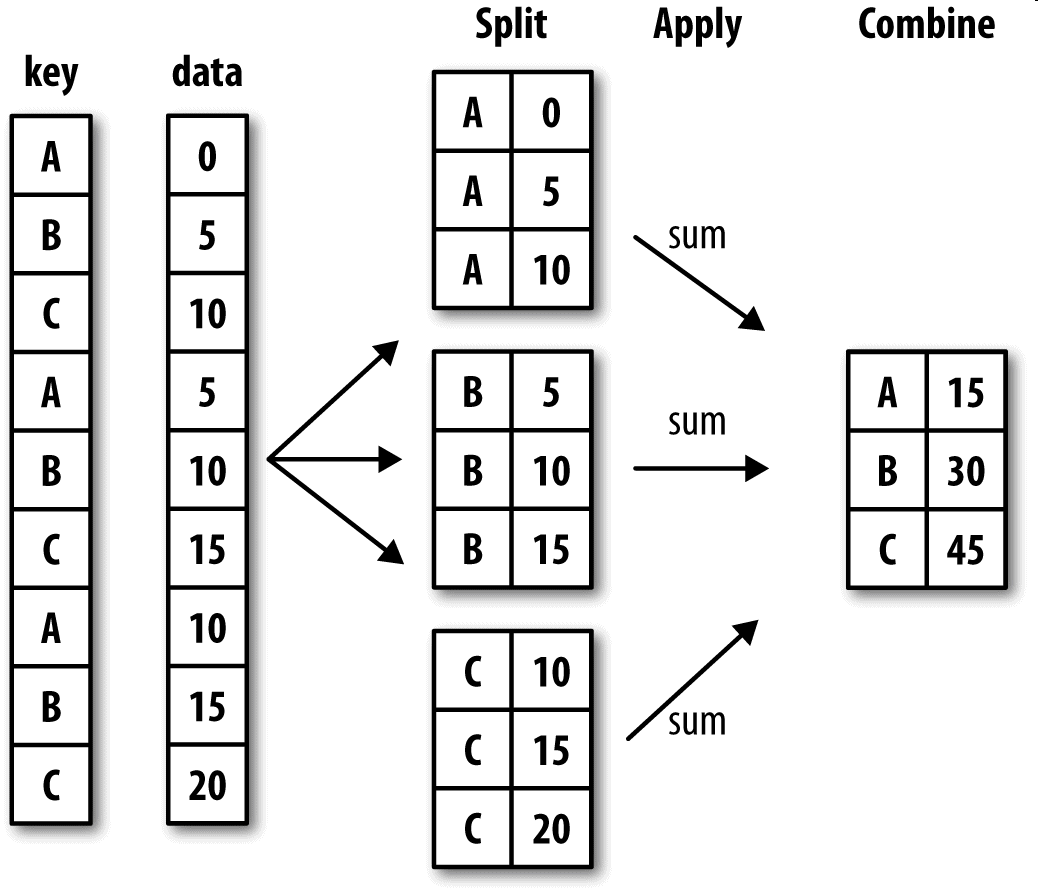分组键可以是多种样式，并且键不一定是完全相同的类型：
列表或者数组，长度要与待分组的轴一样表示DataFrame某个列名的值。字典或Series，给出待分组轴上的值与分组名之间的对应关系函数，用于处理轴索引或者索引中的各个标签
后三种只是快捷方式，最终仍然是为了产生一组用于拆分对象的值。
首先，通过一个很简单的DataFrame数组尝试一下：
df = pd.DataFrame({'key1':['x','x','y','y','x',
'key2':['one','two','one',',two','one'],
'data1':np.random.randn(5),
'data2':np.random.randn(5)})
df

>   key1    key2    data1   data2
0   x   one 0.951762    1.632336
1   x   two -0.369843   0.602261
2   y   one 1.512005    1.331759
3   y   two 1.383214    1.025692
4   x   one -0.475737   -1.182826

访问data1，并根据key1调用groupby：
f1 = df['data1'].groupby(df['key1'])
f1

> <pandas.core.groupby.groupby.SeriesGroupBy object at 0x00000275906596D8>

上述运行是没有进行任何计算的，但是我们想要的中间数据已经拿到了，接下来，就可以调用groupby进行任何计算
f1.mean()  # 调用mean函数求出平均值
> key1
x    0.106183
y    2.895220
Name: data1, dtype: float64

以上数据经过分组键（一个Series数组）进行了聚合，产生了一个新的Series，索引就是key1列中的唯一值。这些索引的名称就为key1。接下来就尝试一次将多个数组的列表传进来
f2 = df['data1'].groupby([df['key1'],df['key2']])
f2.mean()
> key1  key2
x     one     0.083878
two     0.872437
y     one    -0.665888
two    -0.144310
Name: data1, dtype: float64


传入多个数据之后会发现，得到的数据具有一个层次化的索引，key1对应的x\y;key2对应的one\two.
f2.mean().unstack()  # 通过unstack方法就可以让索引不堆叠在一起了

> key2  one     two
key1
x   0.083878    0.872437
y   -0.665888   -0.144310


补充：
1、分组键可以是任意长度的数组2、分组时，对于不是数组数据的列会从结果中排除，例如key1、key2这样的列3、GroupBy的size方法，返回一个含有分组大小的Series
# 以上面的f2测试
f2.size()
> key1  key2
x     one     2
two     1
y     one     1
two     1
Name: data1, dtype: int64

到这跟着我上面的步骤一步一步的分析，会发现还是很简单的，但是一定不能干看，还要自己下手练，只有多练才能融汇贯通！！！3.4.2、聚合(组内应用某个函数)
聚合是指任何能够从数组产生标量值的数据转换过程。刚才上面的操作会发现使用GroupBy并不会直接得到一个显性的结果，而是一个中间数据，可以通过执行类似mean、count、min等计算得出结果，常见的还有一些:
函数名描述sum非NA值的和median非NA值的算术中位数std、var无偏（分母为n-1）标准差和方差prod非NA值的积first、last第一个和最后一个非NA值

自定义聚合函数

不仅可以使用这些常用的聚合运算，还可以自己自定义。
# 使用自定义的聚合函数，需要将其传入aggregate或者agg方法当中

def peak_to_peak(arr):
return arr.max() - arr.min()
f1.aggregate(peak_to_peak)
运行结果：
key1
x    3.378482
y    1.951752
Name: data1, dtype: float64

多函数聚合：
f1.agg(['mean','std'])
运行结果：
mean    std
key1
x   -0.856065   0.554386
y   -0.412916   0.214939

最终得到的列就会以相应的函数命名生成一个DataFrame数组以上我们是可以通过agg或者是aggregate来实现多函数聚合以及自定义聚合函数，但是一定要注意agg方法只能进行聚合操作，进行其他例如：排序，这些方法是会报错的。agg返回的是数据的标量，所以有些时候并不适合使用agg，这就要看我们接下来的操作了。
3.4.3、apply
​ GroupBy当中自由度最高的方法就是apply，它会将待处理的对象拆分为多个片段，然后各个片段分别调用传入的函数，最后将它们组合到一起。

df.apply( ['func', 'axis=0', 'broadcast=None', 'raw=False', 'reduce=None', 'result_type=None', 'args=()', '**kwds']

func:传入一个自定义函数 axis:函数传入参数当axis=1就会把一行数据作为Series的数据
# 分析欧洲杯和欧洲冠军联赛决赛名单
import pandas as pd

url="https://en.wikipedia.org/wiki/List_of_European_Cup_and_UEFA_Champions_League_finals"
a1 = eu_champions    # 取出决赛名单
a1.columns = a1.loc  # 使用第一行的数据替换默认的横向索引
a1.drop(0,inplace=True)  # 将第一行的数据删除
a1.drop('#',axis=1,inplace=True)  # 将以#为列名的那一列删除
a1.columns=['Season', 'Nation', 'Winners', 'Score', 'Runners_up', 'Runners_up_Nation', 'Venue','Attendance']  # 设置列名

a1.tail()  # 查看后五行数据
a1.drop([64,65],inplace=True)  # 删除其中的缺失行以及无用行
a1

运行结果：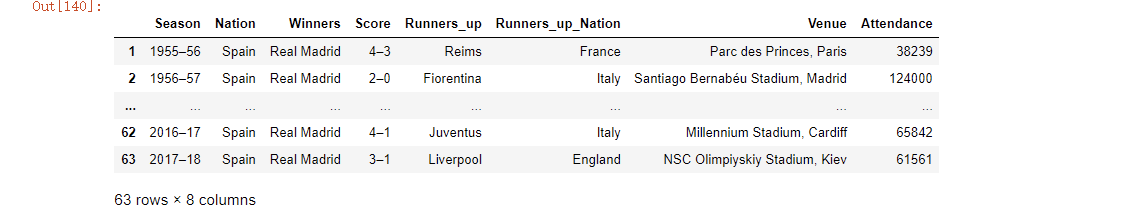现在想根据分组选出Attendance列中值最高的三个。
# 先自定义一个函数
def top(df,n=3,column='Attendance'):
return df.sort_values(by=column)[-n:]
top(a1,n=3)

运行结果：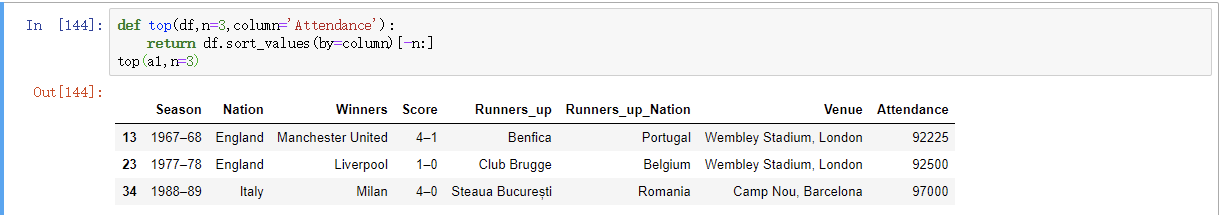接下来，就对a1分组并且使用apply调用该函数：
a1.groupby('Nation').apply(top)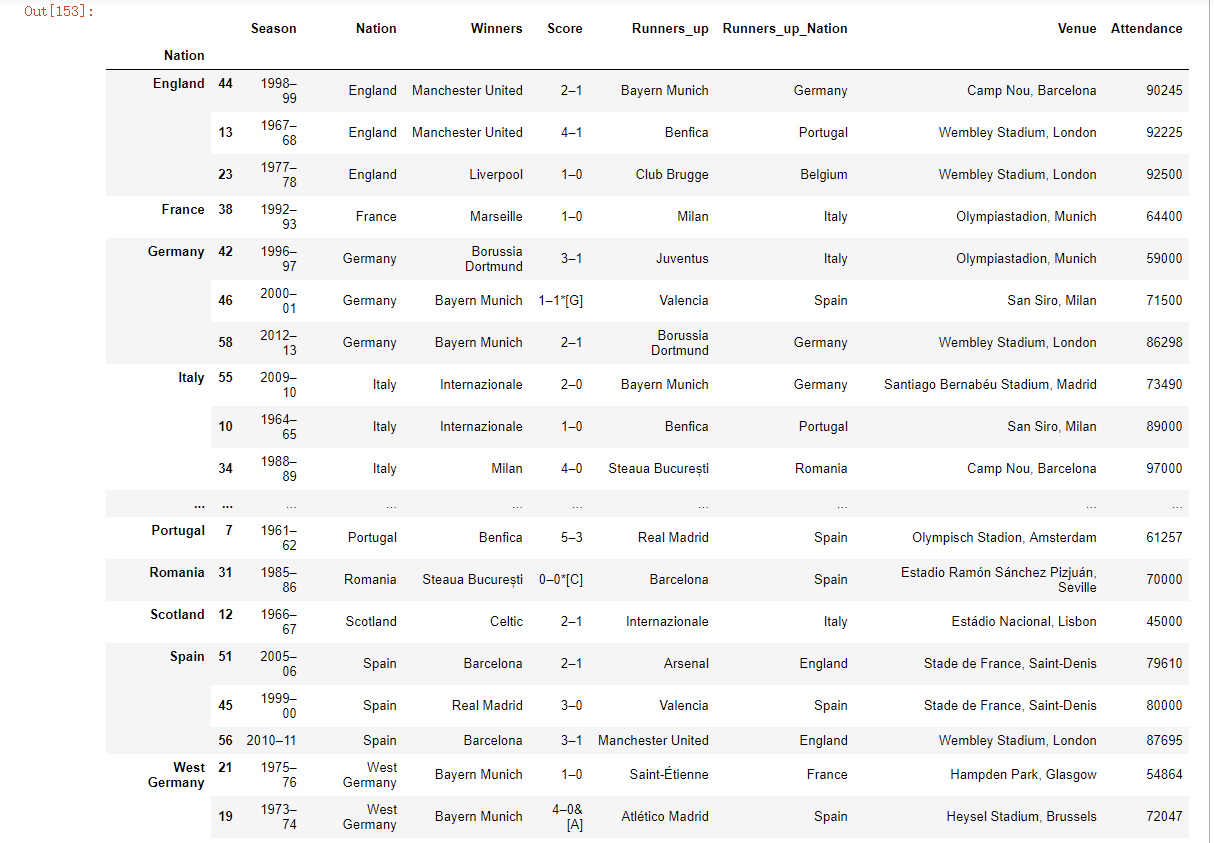运行之后会发现，我们通过这个操作将每个国家各个年份时段出席的人数的前三名进行了一个提取。
以上top函数是在DataFrame的各个片段上调用，然后结果又通过pandas.concat组装到一起，并且以分组名称进行了标记。
以上只是基本用法，apply的强大之处就在于传入函数能做什么都由自己说了算，它只是返回一个pandas对象或者标量值就行3.5、其他常用方法

pandas常用方法（适用Series和DataFrame）

mean(axis=0,skipna=False)sum(axis=1)sort_index(axis, …, ascending) # 按行或列索引排序sort_values(by, axis, ascending) # 按值排序apply(func, axis=0) # 将自定义函数应用在各行或者各列上，func可返回标量或者Seriesapplymap(func) # 将函数应用在DataFrame各个元素上map(func) # 将函数应用在Series各个元素上

转载于:https://www.cnblogs.com/Yang-Sen/p/11061853.html
展开全文• ## pandas

2019-03-14 14:23:05
文章目录Pandas数据处理1....DataFrame本质上是一种带行标签和列标签、支持相同类型数据和缺失值的多维数组。 1. Series对象 Pandas 的 Series对象是一个带索引数据构成的一维数组，可以用一个数组创建Se...
• 文章目录pandas基础pandas介绍pandas核心数据结构SeriesDateTimeIndexDataFrame核心数据结构操作Jupyter notebookpandas核心pandas描述性统计pandas排序pandas分组将数据拆分成组迭代遍历分组获得一个分组细节分组...数据分析
• Pandas进阶贰 pandas基础 pandas进阶系列根据datawhale远昊大佬的joyful pandas教程写一些自己的心得和补充，本文部分引用了原教程，并参考了《利用Python进行数据分析》、numpy官网、pandas官网 为了方便自己回顾和...python 数据分析 大数据
• 总结了这67个pandas函数，完美解决数据处理，拿来即用！python数据处理
• 文章目录Pandas聚合根据一个或多个键拆分pandas对象GroupBy分组键对分组进行迭代通过字典或Series进行分组通过函数分组根据索引级别分组计算分组摘要统计对DataFrame的列应用各种各样的函数应用组内转换或其他运算...
• df_csv = pd.read_csv('G:\代码\joyful-pandas-master\data\my_csv.csv') # 可以写相对路径也可以写绝对路径 df_csv col1 col2 col3 col4 col5 0 2 a 1.4 apple 2020/1/1python 数据分析
• Pandas 入门 两个数据类型：series和DataFrame Series 1. 创建Series对象 import pandas as pd ser1 = pd.Series(data=[], index=[]) 2. Series对象的属性 索引：ser1.index() —> 索引的值： ser1.index....python
• 首先介绍一下numpy 和 pandas的区别： numpy pandas 基础数据类型 扩展数据类型 关注的是数据结构表达 关注的是数据应用表达 维度：数据间的关系 ...
• 第二章 pandas基础 import numpy as np import pandas as pd import xlrd 在开始学习前，请保证pandas的版本号不低于如下所示的版本，否则请务必升级！请确认已经安装了xlrd, xlwt, openpyxl这三个包，其中xlrd版本...python
• 如果有相同索引则进行算术运算，如果没有则会进行数据对齐，但会引入缺失值。对于DataFrame类型，数据对齐的操作会同时发生在行和列上。 import pandas as pd import numpy as np ##Series相加 obj1 = pd.Series([1...python numpy
• Pandas 是Python的核心数据分析支持库，提供了快速、灵活、明确的数据结构，旨在简单、直观地处理关系型、标记型数据。Pandas常用于处理带行列标签的矩阵数据、与 SQL 或 Excel 表类似的表格数据，应用于金融、统计...数据处理
• pandas 一、Series（一维数据）(创建、查找、属性、日期类型数据) Series可以理解为一个一维的数组，只是index名称可以自己改动。类似于定长的有序字典，有Index和 value。 个人理解，Series生成的是行数的一维数组...
• 前言Pandas是机器学习三剑客之一，我们知道Numpy能够对数据进行很好的分析、操作、矩阵计算等，Pandas更侧重于数据的处理和分析，它的底层是使用Numpy实现的，在数据处理和分析方面提供了强大的功能。下面就总结一下......